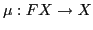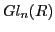# Differential Geometry and Mathematical Physics

### Friday January 22

(the detailed schedule has not been decided yet)

14:00-14:45
Demeter Krupka (Masaryk University, Brno)
The Cartan form and its generalizations in the calculus of variations

We discuss possible extensions of the concept of the Cartan form of classical mechanics to higher order mechanics on manifolds, higher order field theory on jet bundles and to parametric variational problems on slit tangent bundles and on bundles of non-degenerate velocities. We present a generalization of the Cartan form, known as a Lepage form, and basic properties of the Lepage forms. Both earlier and recent examples of differential forms generalizing the Cartan form are reviewed.

14:45-15:30
Eva Miranda (Universitat Politècnica de Catalunya)
Symplectic and Poisson geometry of b-manifolds

In this talk we will try to show the hidden geometry (symplectic and Poisson) in what we call b-manifolds.

These manifolds were initially considered by Nest and Tsygan while studying formal deformations of symplectic manifolds with boundary and also by Melrose in the context of differential calculus and differential operators of manifolds with boundary.

Symplectic b-manifolds lie between the symplectic and Poisson world. In particular, it is possible to prove local and semiglobal normal forms via b-de Rham theory. This talk is based on joink work with Victor Guillemin and Ana Rita Pires.

16:00-16:30
Ján Brajerčík (University of Prešov)
Order reduction of the Euler-Lagrange equations of higher order invariant variational problems on frame bundles

Letbe a principal bundle of frames with the structure group. It is shown that the variational problem, defined by-invariant Lagrangian on Jr FX, can be equivalently studied on the associated space of connections with some compatibility condition, which gives us order reduction of the corresponding Euler-Lagrange equations.

16:30-17:00
Jose Antonio Vallejo Rodriguez (State University of San Luis Potosi, Mexico)
Some generalizations of the notion of Lie algebra

There are several ways to generalize the notion of Lie algebra. For instance, one can lift the restriction of antisymmetry (obtaining the so called Loday -or Leibniz- algebras), or view a particular Lie algebra as a member of a family indexed by the points of a manifold (a kind of moduli space called Lie algebroid). Alan Weinstein has shown how any finite-dimensional Lie algebra can be put in correspondence with a closed maximal subspace of a certain construction called omni-Lie algebra. In the talk, we will describe a way of unifying those concepts through the notion of omni-Loday algebroid, presenting some simple examples.

17:00-17:30
Martin Swaczyna (University of Ostrava)
Example of nonholonomic constraint of the second order

### Monday January 25

9:00-9:45
Olga Krupková (Palacký University, Olomouc)
The nonholonomic variational principle

A variational principle for mechanical systems and fields subject to nonholonomic constraints is found, providing Chetaev-reduced equations as equations for extremals. Two cases are studied: first, when the constrained system arises from an unconstrained Lagrangian system defined in a neighbourhood of the constraint, and second, more general, when an "internal" constrained system on the constraint manifold is given.

9:45-10:30
Xavier Gràcia (Technical University of Catalonia, Barcelona)
Constraints in k-presymplectic field theory

Field theories have several geometric formulations. An especially simple one is the k-symplectic formalism, since only tangent and cotangent bundles are needed in its description. Its defining elements have a close relationship with those in the symplectic formulation of mechanics. The purpose of this talk is to show that this relationship also stands in the presymplectic case, and more particularly to show that one can mimick the presymplectic constraint algorithm to obtain a constraint algorithm that can be applied to k-presymplectic field theory.

11:00-11:30
Artur Sergyeyev (Silesian University, Opava)
Generalized Stäckel transform and its applications to integrable systems

In this work we introduce multiparameter generalized Stäckel transform - a noncanonical transformation of a special kind relating the sets of (not necessarily commuting) Hamiltonians and leaving the phase space coordinates intact. It is shown that under certain conditions the transformation in question preserves Liouville integrability, noncommutative integrability and superintegrability. For instance, when applied to an n-tuple of commuting Hamiltonians, this transformation yields a (new) n-tuple of commuting Hamiltonians. Thus, one can construct new integrable systems from the old ones and also fi nd new links among known integrable systems using the transformation under study. In particular, we show that integrable systems associated with the so-called k-hole deformations of the separation curve of Benenti type are related to the ones associated with the original separation curve of Benenti type through an appropriately chosen multiparameter generalized Stäckel transform. The corresponding transformation for the equations of motion proves to be nothing but a reciprocal transformation of a special form, and we briefly discuss the properties of this class of reciprocal transformations, including applications to hydrodynamic-type systems.

This is joint work with Maciej Blaszak. For more details see A. Sergyeyev, M. Blaszak, Generalized Stäckel transform and reciprocal transformations for finite-dimensional integrable systems, J. Phys. A: Math. Theor. 41 (2008), paper 105205.

11:30-12:00
Vlasta Peřinová (Palacký University, Olomouc)
Maxwell equations with periodic coefficients in 1 + 1 dimensions for an optical application

On using the Fourier transformation, the time included in the Maxwell equations can be replaced by the frequency of a component, but a simplification does not come about, in spite of the parametric approximation, when a field in a nonlinear medium is described. We encounter spatially periodic coefficients. We deal with a decomposition of a solution into the surface and volume components in the case, when the nonlinear media of different coefficients of nonlinearity alternate.

12:00-12:30
Milos Tater (Academy of Sciences, Řež)
Asymptotic zero distributions of polynomials of the Heun equation

We solve the classical (multi)spectral problem for the Heun equation suggested by E. Heine and T. Stieltjes in the 19th century. We study asymptotic behaviour of roots of both Van Vleck and Stieltjes polynomials. Existence of the asymptotic measures is proved and their supports are characterized.